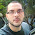## Sunday, 15 April 2012

### Gaussian / Normal Functions in JavaScript

Recently I've been indulging myself writing a small game in JavaScript. It didn't take long before I realised I'd need to use some Gaussian / Normal functions to get what I wanted. Firstly, I wanted my AI players and monsters to behave in a non-deterministic manner while tending towards a given decision. Secondly, I wanted to produce animations with a decent easing function.

The former, I decided, relied upon being able to generate random numbers in JavaScript that are not uniformly distributed (noise), but are normally distributed: being more likely to cluster around a distribution defined by a mean and standard deviation. There is good support for this in Java, but JavaScript only supports the uniform random numbers between 0 and 1.

## Gaussian Random Numbers in JavaScript

You can use the Box Muller Transform to transform two uniform random numbers into two normally distributed random numbers. There is a subtlety about caching the second number for later use, which can be done neatly in JavaScript.

```   /**
* Returns a Gaussian Random Number around a normal distribution defined by the mean
* and standard deviation parameters.
*
* Uses the algorithm used in Java's random class, which in turn comes from
* Donald Knuth's implementation of the Box–Muller transform.
*
* @param {Number} [mean = 0.0] The mean value, default 0.0
* @param {Number} [standardDeviation = 1.0] The standard deviation, default 1.0
* @return {Number} A random number
*/
Math.randomGaussian = function(mean, standardDeviation) {

mean = defaultTo(mean, 0.0);
standardDeviation = defaultTo(standardDeviation, 1.0);

if (Math.randomGaussian.nextGaussian !== undefined) {
var nextGaussian = Math.randomGaussian.nextGaussian;
delete Math.randomGaussian.nextGaussian;
return (nextGaussian * standardDeviation) + mean;
} else {
var v1, v2, s, multiplier;
do {
v1 = 2 * Math.random() - 1; // between -1 and 1
v2 = 2 * Math.random() - 1; // between -1 and 1
s = v1 * v1 + v2 * v2;
} while (s >= 1 || s == 0);
multiplier = Math.sqrt(-2 * Math.log(s) / s);
Math.randomGaussian.nextGaussian = v2 * multiplier;
return (v1 * multiplier * standardDeviation) + mean;
}

};
```

## Gaussian Probability Density Function in JavaScript

The second function here returns a probability density function for a given shape of distribution which I found handy in my animations. By changing the mean and standard deviation, it becomes really easy to make one thing happen after another, and everything is nice and smooth due to the nature of the bell curves. The function below returns a probability density function which returns the value of the distribution at x.

```/**
* Returns a normal probability density function for the given parameters.
* The function will return the probability for given values of X
*
* @param {Number} [mean = 0] The center of the peak, usually at X = 0
* @param {Number} [standardDeviation = 1.0] The width / standard deviation of the peak
* @param {Number} [maxHeight = 1.0] The maximum height of the peak, usually 1
* @returns {Function} A function that will return the value of the distribution at given values of X
*/
Math.getGaussianFunction = function(mean, standardDeviation, maxHeight) {

mean = defaultTo(mean, 0.0);
standardDeviation = defaultTo(standardDeviation, 1.0);
maxHeight = defaultTo(maxHeight, 1.0);

return function getNormal(x) {
return maxHeight * Math.pow(Math.E, -Math.pow(x - mean, 2) / (2 * (standardDeviation * standardDeviation)));
}
};
```

You can find the complete, up-to-date file here on GitHub

#### 2 comments:

1.Hello,is there any javascript/jquery library to generate gussian reports?

2.Certsout.com provides authentic IT Certification exams preparation material guaranteed to make you pass in the first attempt. Download instant free demo & begin preparation. 700-651 Exam Practice Test